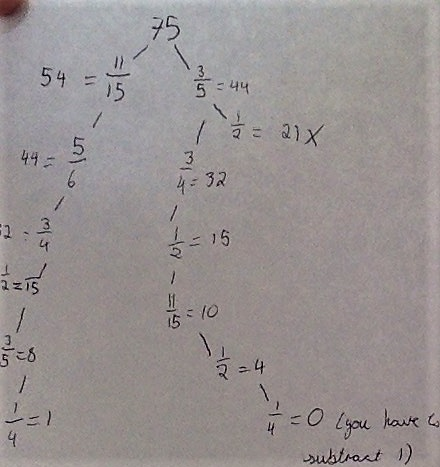#### You may also like### Tweedle Dum and Tweedle Dee

Two brothers were left some money, amounting to an exact number of pounds, to divide between them. DEE undertook the division. "But your heap is larger than mine!" cried DUM...### Sum Equals Product

The sum of the numbers 4 and 1 [1/3] is the same as the product of 4 and 1 [1/3]; that is to say 4 + 1 [1/3] = 4 ï¿½ 1 [1/3]. What other numbers have the sum equal to the product and can this be so for any whole numbers?### Special Sums and Products

Find some examples of pairs of numbers such that their sum is a factor of their product. eg. 4 + 12 = 16 and 4 × 12 = 48 and 16 is a factor of 48.

# Peaches Today, Peaches Tomorrow...

##### Age 11 to 14Challenge Level

(i) 60 peaches
Well done to everyone who worked out how many peaches the monkey was left with. This is Angela's work, from Westridge School in the USA:

1st day: $\frac34 \times 60=45$      $45-1=44$
2nd day: $\frac7{11} \times 44=28$      $28-1=27$
3rd day: $\frac59 \times 27=15$      $15-1=14$
4th day: $\frac27 \times 14=4$        $4-1=3$
5th day: $\frac23 \times 3=2$         $2-1=1$

The monkey had $1$ peach at the end of 5 days.

(ii) 75 peaches
Well done to Anh Minh from British Vietnamese International School Hanoi, Siddhant from Singapore International School in India, Iqra from Uphall Primary School, Robyn and Connie from St Stephen's CE Primary School, EDSR from Priestlands School, Tommy and Sam from Widey Court Primary School and Jessica from Edmund de Moundeford (all in the UK) who put the fractions in the correct order. Saya and Addison from Westridge described their method:

First, we started with 75 peaches (like said in the problem). Then, we looked at all the denominators and wondered which could divide 75 without a remainder. The options were $\frac{11}{15}$ or $\frac35$ because 75 could be divided by their denominator evenly. We decided with $\frac{11}{15}$ because that would give us the largest amount of peaches to keep out of the two. Then, we kept going with this idea to look at the denominators to see which would be the best choice for the next day. The order that we ended up with was $\frac{11}{15},\frac56,\frac34, \frac12, \frac35,$ and then $\frac14.$

Xaviera from Humanitree in Mexico used a slightly different method:
Making a tree diagram helped me a lot. With a tree diagram, one for each fraction, you can rule out numbers that take you to a dead end or numbers that might work if you use them a different way.

Keane and Michael from Bangkok Patana School in Thailand considered which fractions could go at the end:
On the last day, the fraction he wants to keep needs to be one of the unit fractions, or in other words, $\frac12$ or $\frac14$, or a fraction with the numerator as 2. This is because if you have any other fraction, like $\frac35$, and you have a number like 5, the number would be 3, and if you subtract 1, it would still be 2 peaches. But if you had $\frac25$, and the number was 5, the result would be 2, and minus 1 would be 1. Same applies for the unit fractions, but the denominator has to be half the amount starting, so for example, you would have to have 8 for $\frac14$ to work, or 4 for the $\frac12.$

(iii) Making the peaches last as long as possible

7 days: D from HumanitreeEDSR (with more peaches left over to last more days)
8 days: Mateo and Ian from Humanitree
11 days: Shaufie and Xaviera from Humanitree
12 days: Burapa and Jesse from Bagkok Patana School
13 days: Dana and A from Humanitree
14 days: Lorea from Humanitree, Siddhant
15 days: Marcelo  from Humanitree
16 days: Dan from the UK, Keane and Michael

Burapa and Jesse chose their starting number of peaches carefully:
We used the trial and error technique but started off with the numbers under 100 with the most factors which were 60, 72, 84, 96
Since 96 was the biggest number, we thought that it would be able to be the number that could be divided the most.

Most other people used a combination of these ideas decribed by Keane and Michael:
Our method was to find the biggest factor of the number of peaches we had, [which would] be the denominator of the amount of peaches we keep, and use the biggest possible numerator. For instance, 32/33 of 99. Then, we subtract 1. If the fraction makes a prime number after subtracting 1, then we make the numerator 1 less and see if it works then. If is still doesn't work, we keep going with the process. We kept doing that until we could no longer do it.